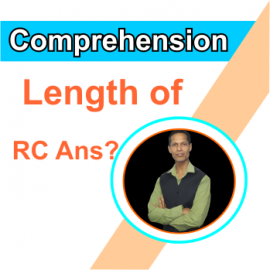# What should be the length of RC answers? |RBI|NABARD|SEBIThis is a frequently asked question ‘what should be the length of the answers to the questions of the given passage?’ In regulatory body exams, there are five questions set each carrying at least 5 marks. In SEBI Grade ‘A’ exam 2021, each question based on the given passage was of 8 marks, thus totaling 40 marks. Thus, raising doubts about the length of the answers is quite natural among the aspirants.

There are mainly two factors that decide the length of the answers, which are:

The allocation of marks for each question as the more weightage of marks is there, the more elaboration on the answers is needed.

The nature of the questions